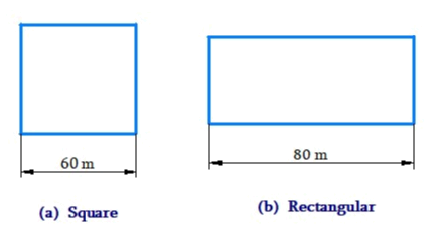# Ex.11.1 Q1 Mensuration Solutions - NCERT Maths Class 8

Go back to  'Ex.11.1'

## Question

A square and a rectangular field with measurements as given in the figure have the same perimeter. Which field has a larger area?

Video Solution
Mensuration
Ex 11.1 | Question 1

## Text Solution

What is Known?

Perimeter of rectangular field and square area same and also one side of square and rectangular field are known.

What is unknown?

Area of square and rectangular field.Reasoning:

It is given that perimeter of the square and the rectangular field are same, so we can find breadth of the rectangular field and also the area of the rectangular field.

Steps:

Side of the square $$= 60\rm\,m$$

Length of the rectangle $$= 80\rm\,m$$

Perimeter of the square \begin{align} &= 4 \times {\text{side of square}}\\ &= 4 \times 60 {\rm{m}} = 240 {\rm{m}} \end{align}

Perimeter of rectangle $$= 2 \times ({\rm{length}} + {\rm{breadth}})$$

Perimeter of square $$=$$ Perimeter of rectangle

\begin{align}240 &= \! 2\! \times \!( \! {\rm{length}} \!+\! {\rm{breadth}}\!)\\240 &= 2 \times (80 + {\rm{breadth}})\\240 &= 160 + 2 \times {\rm{breadth}}\\240 - 160 &= 2 \times {\rm{breadth}}\\{\rm{80 }}&= 2 \times {\rm{breadth}}\end{align}

${\rm{breadth}} = \frac{{80}}{2} = 40\rm\,m$

Area of the square

\begin{align} &= {\rm{side}} \times {\rm{side}} \\ &= 60 \times 60 \\ &= 3600{{\rm{m}}^2}\end{align}

Area of the rectangular field

\begin{align} &= {\rm{length}} \times {\rm{breadth}}\\ &= 80 \times 40 = 3200{{\rm{m}}^2}\end{align}

Thus, area of square is larger than the area of rectangular field.

Learn from the best math teachers and top your exams

• Live one on one classroom and doubt clearing
• Practice worksheets in and after class for conceptual clarity
• Personalized curriculum to keep up with school